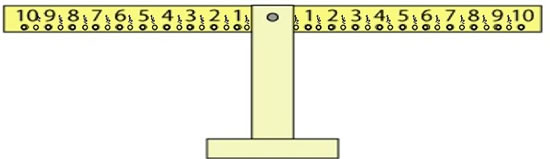#### You may also like### Cubes

How many faces can you see when you arrange these three cubes in different ways?### Pebbles

Place four pebbles on the sand in the form of a square. Keep adding as few pebbles as necessary to double the area. How many extra pebbles are added each time?### Bracelets

Investigate the different shaped bracelets you could make from 18 different spherical beads. How do they compare if you use 24 beads?

# Balance of Halves

## Balance of Halves

Before you try this activity, you might like to have a go at Number Balance and Getting the Balance, if you haven't already.

Here is a balance, or equaliser, which you might like to investigate:

Full Screen Version

Now, what about a balance that has halves on it?Imagine we hung a weight on the number 7 on the left-hand side. Let's say we can only use the "halves" on the right. Which two numbers on the right-hand side could you hang weights from so that it would balance?

For example, you might have a weight on the $\frac{1}{2}$ and the $6\frac{1}{2}$.

Where else could you hang two weights on the right-hand side to make it balanced?

You can set yourselves all kinds of problems with this new set-up.
Or, "I wonder what it'd be like to have three weights balancing two using halves wherever we wanted?"

Let us know what you find out.

### Why do this problem?

Whether this problem is done with a balance or with pencil and paper, there is more to it than just doing addition. Although addition and subtraction are involved, you are likely to find the children using all sorts of language associated with these two operations, and one of the main points here is to establish a balance or equivalence as well as "equals". Some pupils are not so readily used to coming across situations like $3 + 5 = 6 + 2$, for example. You could use this activity to introduce some algebraic ideas.

### Possible approach

You may encourage the class to articulate what they are trying to find in a general sense. For example "$7$ balances two other numbers", or "$7$ = 'something' add 'something'"or "$7$ = ? $+$ ?". This will help them to get the idea that they are finding different numbers which fit this criterion - not just one answer.

### Key questions

How might you start?
How might you record your thinking?
How do you know you've got all the possibilities?

### Possible extension

As the question suggests, some learners might like to look at a balance involving other fractions.

### Possible support

Children might like to try Getting the Balance instead.